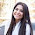## Friday, May 30, 2014

### XAT 2014 Solved Question Paper : Video Solutions.

Today we look at a few questions that appeared in XAT 2014 exam. Note that XAT 2014 questions are also a good set of practice questions for CAT 2014.

The questions below have the question followed by a Video Solution!

1.Evaluate the following:
The solution to this problem is explained in the Video below:

2. Two numbers, 297B and 792B, belong to base B number system. If the first number is a factor of the second number then the value of B is

11
12
15
17
19

3. Aditya has a total of 18 red and blue marbles in two bags (each bag has marbles of both colors). A marble is randomly drawn from the first bag followed by another randomly drawn from the second bag, the probability of both being red is 5/16. What is the probability of both marbles being blue?

4. The probability that a randomly chosen positive divisor of 1029 is an integer multiple of 1023 is a2/b2, then 'b - a' would be:

8
15
21
23
45

5. The number of the possible values of X in the equation |X + 7| + |X - 8| = 16 is

0
1
2
3
4

6. A polynomial ax3 + bx2 + cx + d intersects x-axis at 1 and -1, and y-axis at 2. The value of b is:

-2
0
1
2
Cannot be Determined

7. S = ax/(b+c+x) where all parameters are positive. If x is increased and all other terms are kept constant, S will

Increase
Decrease
Increases and then decreases
Decreases and then increases
Cannot be determined

8. x, 17, 3x - y2 - 2, and 3x + y2 - 30, are four consecutive terms of an increasing arithmetic sequence. The sum of the four number is divisible by:

2
3
5
7
11

In our future posts, we will be adding more questions from XAT 2014, which should be also be a very good practice set for CAT 2014.

1.2.This is an interesting and the most possessive topic for all the new consumer because the most famous Proton Pakistan is giving their most beneficial SEDAN cars for sale in Faisalabad because to improving the economy experiences in Pakistan.

3.With the exteriors of the two smithereens of fabric facing each other, sew about three sides of the bags & cases, preliminary at one lateral, to the lowest and back along the additional side, sendoff the top open.

4.Thanks for sharing such question papers, This will help candidates to prepare banking exams like RBI Grade B, NABARD Grade A Exams.

5.6.